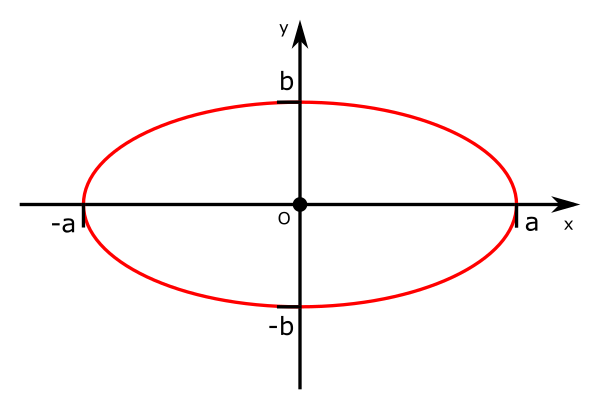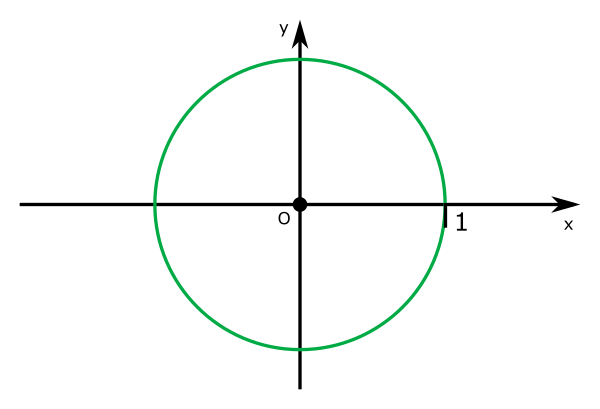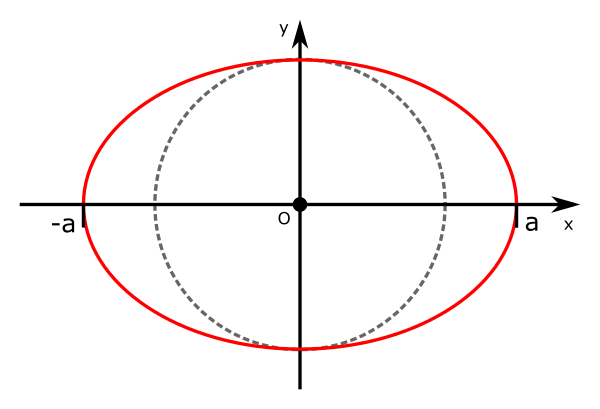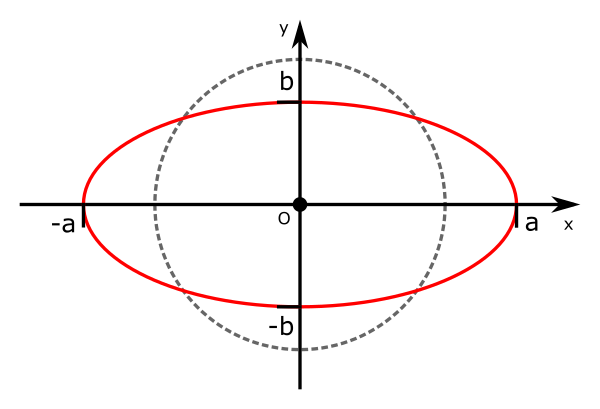# Parametric equation of ellipse

Martin McBride, 2020-09-14
Tags ellipse major axis minor axis
Categories coordinate systems pure mathematics

The parametric equation of an ellipse is:

\begin{align} x = a \cos{t}\newline y = b \sin{t} \end{align}## Understanding the equations

We know that the equations for a point on the unit circle is:

\begin{align} x = \cos{t}\newline y = \sin{t} \end{align}Multiplying the $x$ formula by $a$ scales the shape in the x direction, so that is the required width (crossing the x axis at $x = a$). In this example, $a > 1$ so the circle is stretched in the x direction:Multiplying the $y$ formula by $b$ scales the shape in the y direction, so that is the required height (crossing the y axis at $y= b$). In this example, $b < 1$ so the circle is compressed in the y direction: